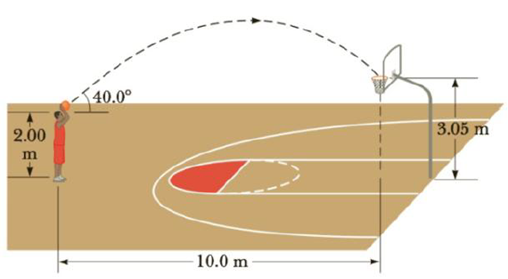Chapter 3, Problem 44AP

Chapter
Section
Textbook Problem

A 2.00-m-tall basketball player is standing on the floor 10.0 m from the basket, as in Figure P3.44. If he shoots the ball at a 40.0° angle with the horizontal, at what initial speed must he throw the basketball so that it goes through the hoop without striking the backboard? The height of the basket is 3.05 m.Figure P3.44

To determine
The initial speed of the basketball.

Explanation

The horizontal component of the initial velocity when the launch angle is 40.0° is,

v0x=v0cos40.0°=0.766v0

The time required for the ball to move 10.0m horizontally is,

t=Δxv0x

Here,

Δx is the horizontal displacement

v0x is the horizontal component of the initial velocity

t=10.0m0.766v0=13.1mv0

The vertical displacement is,

Δy=yy0=3.05m2.00m=1.05m

The equation for vertical displacement is,

Δy=v0yt+12ayt2=(v0sinθ)(13.1mv0)+12ay(13

Still sussing out bartleby?

Check out a sample textbook solution.

See a sample solution

The Solution to Your Study Problems

Bartleby provides explanations to thousands of textbook problems written by our experts, many with advanced degrees!

Get Started